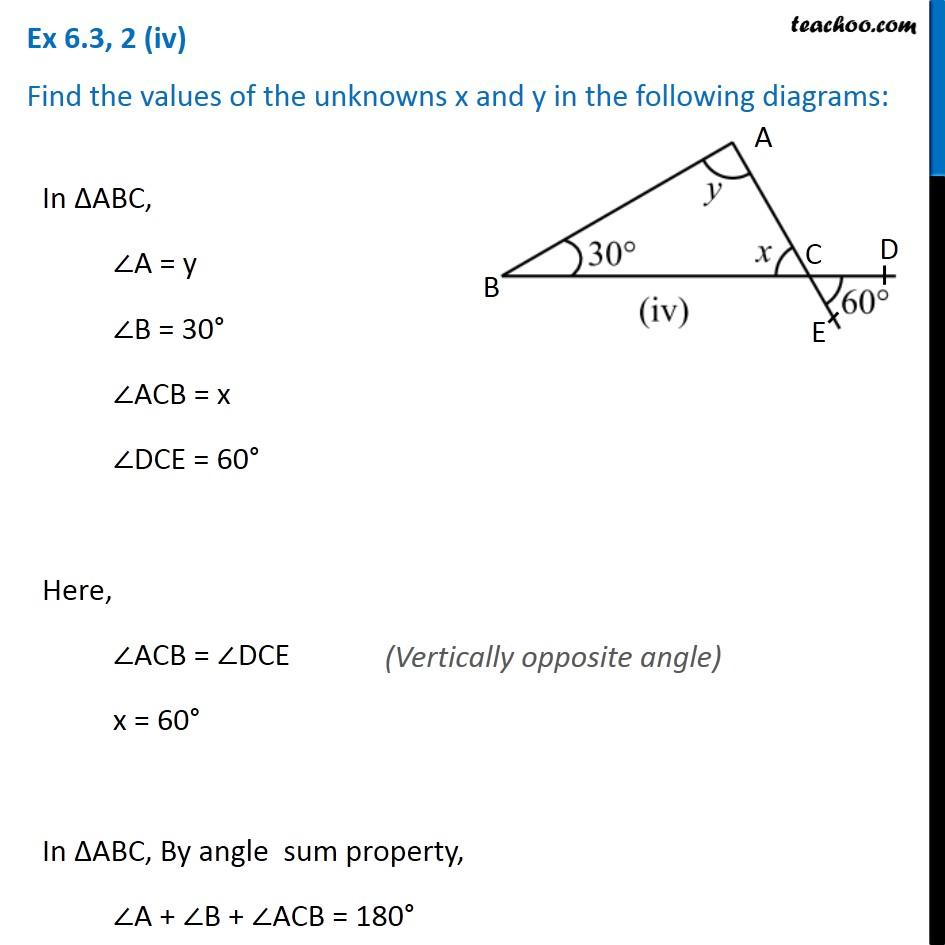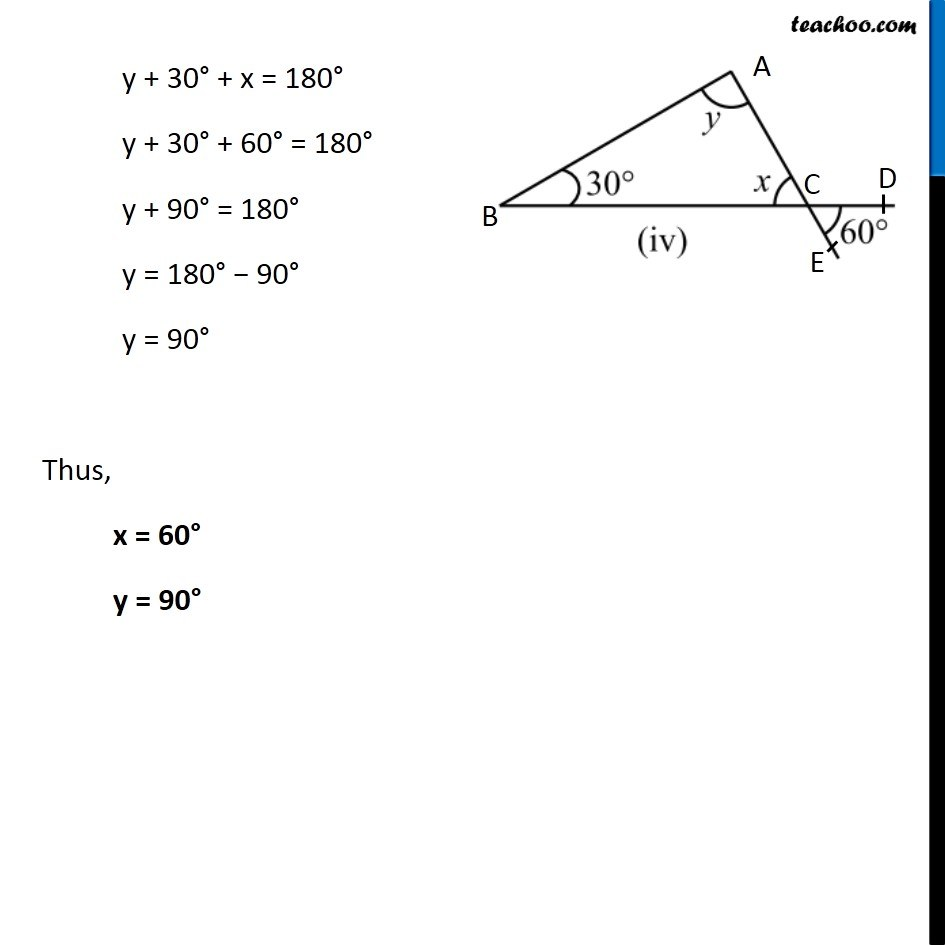Ex 6.3

Chapter 6 Class 7 Triangle and its Properties
Serial order wiseLearn in your speed, with individual attention - Teachoo Maths 1-on-1 Class

### Transcript

Ex 6.3, 2 (iv) Find the values of the unknowns x and y in the following diagrams: In ∆ABC, ∠A = y ∠B = 30° ∠ACB = x ∠DCE = 60° Here, ∠ACB = ∠DCE x = 60° In ∆ABC, By angle sum property, ∠A + ∠B + ∠ACB = 180° y + 30° + x = 180° y + 30° + 60° = 180° y + 90° = 180° y = 180° − 90° y = 90° Thus, x = 60° y = 90°